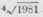The angle of flexure by torsion is as the length and extensibility of the body directly and inversely as the diameter; hence, the length of a bar or shaft being given, the power, and the leverage the power acts with, being known, and also the number of degrees of torsion that will not affect the action of the machine, to determine the diameter in cast iron with a given angle of flexure.

Rule. - Multiply the power in lbs. by the length of the shaft in feet, and by the leverage in feet; divide the product by fifty-five times the number of degrees in the angle of torsion; and the fourth root of the quotient equal the shaft's diameter in inches.

Ex. Required the diameters for a series of shafts 35 feet in length, and to transmit a power equal to 1245 lbs., acting at the circumference of a wheel 2½ feet radius so that the twist of the shafts on the application of the power may not exceed one degree.

1245 X 35 X 2.5 / 55 X 1 =-= 6.67 inches in diameter.

To determine the side of a square, shaft to resist torsion with a given flexure. Bulk. - Multiply the power in pounds by the leverage it acts with in feet, and also by the length of the shaft in feet; divide this product by 92.5 tunes the angle of flexure in degrees, and the square root of the quotient equals the area of the shaft in inches.

Ex. Suppose the length of a shaft to be 12 feet, and to be driven by a power equal tip 700 lbs., acting at 1 foot from the centre of the shaft - required the area of cross section, so that it may not exceed 1 degree of flexure.

700 X 1 X 12 / 92.5 X 1 == 9.53 inches.

Relative strength of Undies to resist Torsion, Lead being 1.

 Tin................ 1.4 Gun Metal.... 5 English Iron...... 10.1 Copper........ 4.3 Cast Iron.... 9 Blistered Steel..... 16.6 Yellow Brass..... 4 6 Swedish Iron 9.5 Shear Steel..... 17